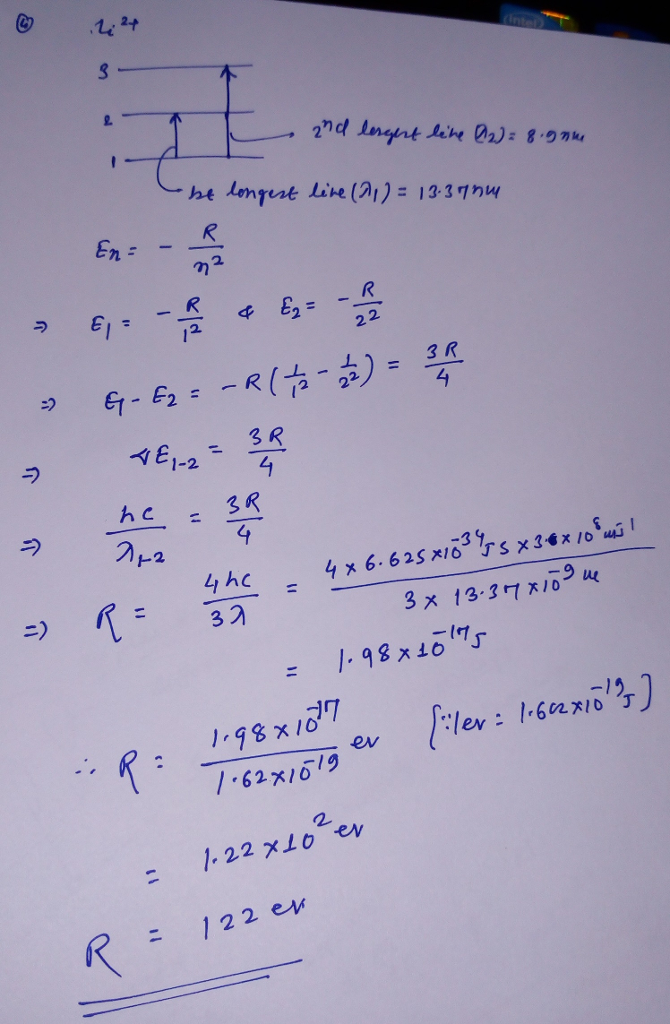# Question & Answer: A gas of doubly ionized lithium atoms (Li2+) has its two longest wavelength absorption…..

A gas of doubly ionized lithium atoms (Li2+) has its two longest wavelength absorption lines at λ1 = 13.37 nm and λ2 = 8.9 nm. If the energy levels are given by

En = −R (1/n2 ), n = 1, 2, 3, . . .

Don't use plagiarized sources. Get Your Custom Essay on
Question & Answer: A gas of doubly ionized lithium atoms (Li2+) has its two longest wavelength absorption…..
GET AN ESSAY WRITTEN FOR YOU FROM AS LOW AS \$13/PAGE

What is the value of the constant R? State your answer in electronvolts (eV).